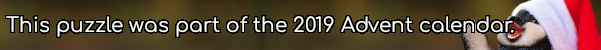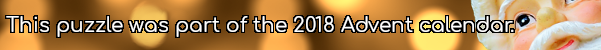mscroggs.co.uk
mscroggs.co.uksubscribe

# Puzzles

## 2 DecemberYou have 15 sticks of length 1cm, 2cm, ..., 15cm (one of each length). How many triangles can you make by picking three sticks and joining their ends?
Note: Three sticks (eg 1, 2 and 3) lying on top of each other does not count as a triangle.
Note: Rotations and reflections are counted as the same triangle.

## 1 DecemberIf you write out the numbers from 1 to 1000 (inclusive), how many times will you write the digit 1?

## Not Roman numerals

The letters $$I$$, $$V$$ and $$X$$ each represent a different digit from 1 to 9. If
$$VI\times X=VVV,$$
what are $$I$$, $$V$$ and $$X$$?

## 24 December1,0,2,0,1,1
The sequence of six numbers above has two properties:
1. Each number is either 0, 1 or 2.
2. Each pair of consecutive numbers adds to (strictly) less than 3.
Today's number is the number of sequences of six numbers with these two properties
Tags: numbers

## 22 DecemberIn base 2, 1/24 is 0.0000101010101010101010101010...
In base 3, 1/24 is 0.0010101010101010101010101010...
In base 4, 1/24 is 0.0022222222222222222222222222...
In base 5, 1/24 is 0.0101010101010101010101010101...
In base 6, 1/24 is 0.013.
Therefore base 6 is the lowest base in which 1/24 has a finite number of digits.
Today's number is the smallest base in which 1/10890 has a finite number of digits.
Note: 1/24 always represents 1 divided by twenty-four (ie the 24 is written in decimal).

## 21 DecemberPut the digits 1 to 9 (using each digit exactly once) in the boxes so that the sums are correct. The sums should be read left to right and top to bottom ignoring the usual order of operations. For example, 4+3×2 is 14, not 10. Today's number is the smallest number you can make using the digits in the red boxes.
 + ÷ = 2 × + - × - = 31 + + - - × = 42 =37 =13 =-2
Tags: numbers, grids

## 20 DecemberToday's number is the sum of all the numbers less than 40 that are not factors of 40.

## 17 DecemberFor $$x$$ and $$y$$ between 1 and 9 (including 1 and 9), I write a number at the co-ordinate $$(x,y)$$: if $$x\lt y$$, I write $$x$$; if not, I write $$y$$.
Today's number is the sum of the 81 numbers that I have written.
Tags: numbers

## Archive

Show me a random puzzle
▼ show ▼